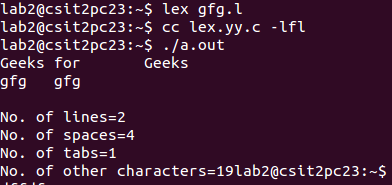# Lex program to count the number of lines, spaces and tabs

• Difficulty Level : Basic
• Last Updated : 22 Mar, 2021

Lex is a computer program that generates lexical analyzers and was written by Mike Lesk and Eric Schmidt. Lex reads an input stream specifying the lexical analyzer and outputs source code implementing the lexer in the C programming language.
Let’s the how to count the number of lines, spaces and tabs using Lex.
Example:

```Input:
Geeks for       Geeks
gfg  gfg

Output:
No. of lines=2
No. of spaces=3
No. of tabs=1
No. of other characters=19

Input:
Hello
How    are    you?

Output:
No. of lines=2
No. of spaces=4
No. of tabs=1
No. of other characters=15```

Below is the implementation:

## C

 `/*lex code to count the number of lines,``    ``tabs and spaces used in the input*/` `%{``#include``int` `lc=0, sc=0, tc=0, ch=0; ``/*Global variables*/``%}` `/*Rule Section*/``%%``\n lc++; ``//line counter``([ ])+ sc++; ``//space counter``\t tc++; ``//tab counter``. ch++;     ``//characters counter``%%` `int` `main()``{``    ``// The function that starts the analysis``    ``yylex();``    ` `    ``printf``(``"\nNo. of lines=%d"``, lc); ``    ``printf``(``"\nNo. of spaces=%d"``, sc);``    ``printf``(``"\nNo. of tabs=%d"``, tc);``    ``printf``(``"\nNo. of other characters=%d"``, ch);``    ` `}`

Output:My Personal Notes arrow_drop_up